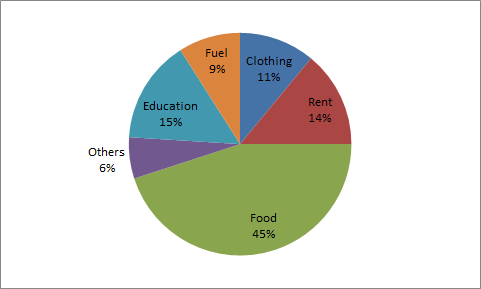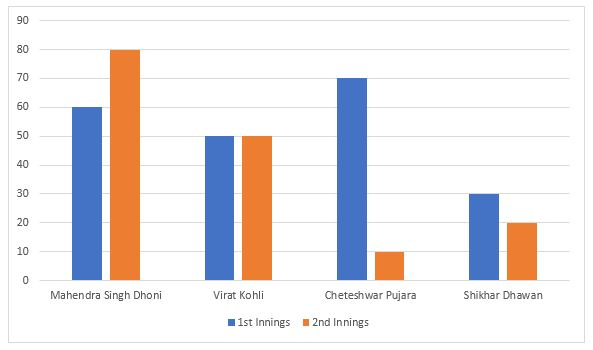# SSC Averages and Percentages Questions with Answers

#### Averages and Percentages

An excellent collection of SSC Averages and percentages questions and answers with detailed explanations for competitive exams. Given below are some of the most repeated practice questions on Averages and percentages for SSC CGL, CHSL, JE, GD constable, Stenographer, MTS, and CPO exams. Go through this very important online quiz based on model and previous year asked questions from SSC Averages and percentages with solutions to ace the exam.

### Take a free SSC CHSL Mock

Thousands of students have taken Cracku's Free SSC CHSL Mock.

Instructions

For the following questions answer them individually

Question 1

Question 2

Question 3

# The average weight of two players P and Q of a football team is 38 kg. The average weight of P, Q and their coach T is 49 kg. What is the weight (in kg) of coach?

Instructions

Directions:The pie chart given below show the spending of a family on various heads during a month. Study the graph and answer the questions.Question 4

Question 5

# Which three expenditures together have a central angle of 108o?

Instructions

For the following questions answer them individually

Question 6

Question 7

Question 8

Question 9

Question 10

Question 11

Question 12

Question 13

Question 14

Question 15

Question 16

Question 17

Question 18

Question 19

Question 20

Question 21

Question 22

Question 23

Question 24

Question 25

Question 26

Question 27

Question 28

Question 29

Question 30

Question 31

Question 32

Question 33

Question 34

Question 35

Question 36

Question 37

Question 38

Question 39

Question 40

Question 41

Question 42

# If A's income is 50% less than that of B's, then B's income is what per cent more than that of A?

Instructions

Given here is a multiple bar diagram of the scores of four players in two innings. Study the diagram and answer the questions:Question 43

# The average runs in two innings of the player who has scored minimum in the second innings are :

Instructions

For the following questions answer them individually

Question 44

Question 45

Question 46

Question 47

Question 48

Question 49

Question 50

Question 51

# If 90% of A = 30% of B and B = x% of A, then the value of x is

Instructions

Directions:The pie chart given below show the spending of a family on various heads during a month. Study the graph and answer the questions.Question 52

# Expenditure on Rent is what percent of expenditure of Fuel?

Instructions

For the following questions answer them individually

Question 53

Question 54

Question 55

Question 56

Question 57